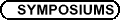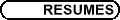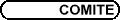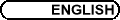Interactions between Algebra and Computer Science / Intéractions entre la science informatique et l'algèbre
Org: Olga Kharlampovich (McGill), Alexei G. Myasnikov (McGill) and/et Vladimir Shpilrain (CUNY)

PETER ACKERMANN, Dortmund University
Gröbner Basis Cryptosystems

We extend and generalize Gröbner basis theory to submodules of free right modules over monoid rings. We then show how this theory can be used to introduce a new class of Gröbner basis cryptosystems and how one can connect these to classical well known cryptosystems.

Geometric Key Establishment

The aim of the talk is to present a new class of key establishment schemes which are based on geometric generalizations of the classical Diffie-Hellman. The simplest of our schemes-based on the geometry of the unit circle-uses only multiplication of rational numbers by integers and addition of rational numbers in its key creation. Preliminary estimations show that our schemes are resistant to attacks. This resistance follows the pattern of the discrete logarithm problem and hardness of multidimensional lattice problems.

ANDREW DUNCAN, Newcastle University
Quantum computation in finite groups: extending the Deutsch-Jozsa-Høyer algorithm

This talk reports a generalisation of the quantum algorithm of the title. One reason for interest in this algorithm is complexity-theoretic: it may help to show whether or not the class of bounded error probabilistic quantum problems BQP is, relative to some oracle, contained in the class of problems in the polynomial hierarchy PH, or not.

Let f be a function f : {0,1}n ® {0,1}. We say f is balanced if |f-1(0)| = |f-1(1)| = 2n-1. Given that f is known either to be a constant function or a balanced function the Deutsch-Jozsa algorithm can determine which: in a single call to a quantum black-box which evaluates the function. By contrast a classical algorithm would need, in the worst case, at least 2n-1+1 evaluations of the function f to perform the same task.

Høyer has shown how this quantum algorithm generalises to functions f : G® H, where G and H are finite groups and f is known to be a function of one of two particular types. In this talk we show how to extend the types of function the algorithm can cope with to a much wider class. Conditions r-balanced and r-constant, on such functions f, are defined which depend on choices of columns of the matrices of the irreducible (unitary) representations of H. We show that we can distinguish between r-balanced and r-constant functions in one call to a quantum black-box for f. In the case where H is cyclic this condition also has a characterisation in terms of cyclotomic polynomials, which will be described.

If time allows the possible complexity theory implications of this generalisation will be discussed.

BEN FINE, Fairfield University, Fairfield, CT 06430
Some Suggestions in Algebraic Cryptography

Because of the increased power of computers the security of cryptosystems, especially public key cryptosystems, is being reexamined. To develop more secure cryptosystems various encryption methods using combinatorial group theory, such as Anshel-Goldfeld and Ko-Lee, have been developed. However several security problems have been pointed out relative to these methods, so that additional work is necessary. In this talk we present some further suggestions for cryptosystems, both public key and classical, based on the use of different representations of free groups. The decryption methods depend upon on group theoretic rewriting systems, such as Reidemeister-Schreier. The difficulty of determining Shreier transversals as well as the introduction of some random "noise" provides the one-way function necessary for a secure cryptosystem. Several experiments based on the computing feasibility of these suggestions will also be mentioned.

This is joint work with G. Baumslag and X. Xu.

LOTHAR GERRITZEN, University of Bochum, D-44780 Bochum, NA 2/33
Planar Calculus

A planar monomial is by definition an isomorphism class of a finite, planar, reduced rooted tree. On the set P of planar monomials there are m-ary grafting operations for m greater or equal to 2. A planar polynomial over a field K is a K-linear combination over P. A planar power series is an infinite formal expression in P over K. If x denotes the tree with a single vertex, any planar power series is an infinite sum over K-multiples of non-associative products in x. The system of m-ary multiplications turn the K-vectorspace A of planar power series into an algebra over the operand freely generated by a sequence of m-ary operations for m > 1. One can substitute g(x) in A for x in f(x) in A to obtain a planar power series f ( g(x) ) in case the order of g(x) is > 0. The derivative d/dx is a derivation on A with d/dx(x) = 1.

There exists a unique planar power series Exp(k,x) in A for any k > 1 such that the order of Exp(k,x) - 1 - x is > 1 and that the k-th power of Exp(k,x) is equal to Exp(k,k*x). Then one can show that d/dx ( Exp(k,x) ) = Exp(k,x). It is called the k-ary planar exponential series. Its coefficients depend algebraically on k. There are also planar series Log(k,x), Sin(k,x), ArcSin(k,x) etc. One can define planar differentials and one can prove a planar chain rule. These examples are instances of a theory which can be called planar calculus. One can expect applications to number theory and cominatorics.

DENNIS HOFHEINZ, Universität Karlsruhe, Fakultät für Informatik, Am Fasanengarten 5, 76128 Karlsruhe, Germany
From hard problems to cryptographic security

Often, the security of a cryptographic system is reduced to a mathematical problem. However, there are various notions of security for, say, a public key cryptosystem, and it is not always clear what notion of security is implied by the hardness of a given mathematical problem. Assuming that factoring of integers is hard, what can we say about the security of plain RSA?

This talk uses the example of cryptography based on braid groups to illustrate in which different ways a mathematical problem can be used for cryptography and what the respective consequences are. In particular, several variations of problems in braid groups are related to security properties of public key encryption and signature schemes.

STEPAN HOLUB, Charles University, Sokolovska 83, Prague
Binary GPCP is in PTIME

Post Correspondence Problem (PCP) consists in determining whether for two morphisms g,h : A*® B*, the equality g(w)=h(w) can be obtained for some non-empty word w Î A*. The input of the generalized version of the problem (GPCP) contains in addition to morphisms g and h words p1, p2, s1, s2, and asks whether there is a word w such that p1 g(w)s1=p2 h(w)s2.

The importance of the problem is given by the fact that it is one of the most famous undecidable problems, reduction to which has been used to prove undecidability of other problems. There are however special cases, in which the problem is decidable. For example, in the binary case, i.e., if the cardinality of A is two, GPCP is well known to be decidable. The decision algorithm described in the literature admits exponential complexity.

With help of an additional insight into the structure of the solutions we show that binary GPCP is in PTIME.

JOINT PANEL DISCUSSION
Participating Sessions

This is a joint Panel Discussion of the following sessions:

• Interactions between Algebra and Computer Science

• Combinatorial and Geometric Group Theory

• Groups, Equations, Non-commutative Algebraic Geometry

ILYA KAPOVICH, University of Illinois at Urbana-Champaign

We investigate the consequences of Kingman's Subadditive Ergodic Theorem for interpreting the results of various group-theoretic experiments. This applies to generic subgroup distortion, random elements in regular languages, the behaviour of various normal forms algorithms, etc. The talk is based on a portion of a joint paper with Paul Schupp and Vadim Kaimanovich.

BILAL KHAN, John Jay College (CUNY), 899 Tenth Avenue, New York, NY
On positive theories of fully residually free groups

Let f be a standard-form first-order sentence in the language of group theory extended by constants from a group G. Specifically, take

 f: "x1 \$y1 "x2 \$y2 ¼"xk \$yk  f0 (x1, y1, x2, y2, ..., xk, yk),
where f0 is a quantifier-free conjunction of disjunctions of equations and inequalities.

We say that f lifts in G, if G \models f and witness values for each existentially quantified variable yi are expressible as elements of G[X] = G*F(X), i.e., there is a Skolemization of f using elements of G[X].

The sentence f is called positive if it is logically equivalent to a standard-form sentence in which f0 contains no inequalities. The set of all positive sentences that are true in G is called the positive theory of G.

The seminal result in this area is due to Merzlykov who showed (though not in this terminology) that if G is a free non-abelian group, then the positive theory of G lifts. We extend Merzlykov's result to a large class of finitely generated fully residually free groups, including surface groups, extensions of centralizers, and others.

This is joint work with Alexei G. Miasnikov and Denis Serbin.

MARTIN KREUZER, Fachbereich Mathematik, Universitaet Dortmund, D-44221 Dortmund, Germany
Some Applications of Groebner Bases to Group Theory

Given a finitely presented group G, we construct the universal representation F: G ® Matn(R) where R = K[x1,...,xn]/I is an affine algebra. Based on Groebner basis computations for ideals in R, we can solve the word problem, the subgroup problem, etc., for the image F(G). In particular, using a new algorithm for computing minimal polynomials of elements in non-commutative algebras, we can show that some elements of F(G) have finite resp. infinite order. This yields a new approach to checking the finiteness of G computationally. Moreover, we shall see examples where Groebner basis computations lead to new insights into the structure of the group G.

STUART MARGOLIS, Bar-Ilan University, Queens College (NY) and Hunter College
Representation Theory and the Theory of Automata and Regular Languages

Representation theory is one of the most classical areas of finite semigroup theory. It was developed by Clifford, Munn, Ponizovsky and others in the 1950s and has been resurrected by Putcha, Renner and Okninski and their students in the last 15 years. On the other hand, the main thrust of research in finite semigroups for the last 40 years has been its connection with the theory of automata and formal languages following the Krohn-Rhodes Theorem and the theory of pseudovarieties developed originally by Eilenberg and Schutzenberger.

Up to now, except for some work of Rhodes in the 1960s, there has been almost no use of representation theory in the automata theoretic aspects of finite semigroup theory. In this talk, we show that there are some very deep connections between the two fields. We show that the collection of finite semigroups triangularizable and unitriangularizable over a finite field forms a pseudovariety and give both a syntactic and semantic description of this collection. We also generalize a Theorem of Rhodes by calculating the congruence obtained by restricting the Jacobson radical of the semigroup algebra KS to S for all fields K and all finite semigroups S. Finally, we show a strong relationship between the language theoretic operation of counting subwords over a field K to the irreducible representations of the syntactic monoid M(L) of languages L over the field K.

This is joint work of Jorge Almeida, Benjamin Steinberg and Mikhail Volkov.

ALEX D. MIASNIKOV, The Graduate Center of CUNY
Heuristics for The Whitehead Minimization Problem

In this talk we will discuss several heuristic strategies which allow one to solve the Whitehead's minimization problem much faster (on most inputs) than the classical Whitehead algorithm. The mere fact that these strategies work in practice leads to several interesting mathematical conjectures. In particular, we conjecture that the length of most non-minimal elements in a free group can be reduced by a Nielsen automorphism which can be identified by inspecting the structure of the corresponding Whitehead Graph.

GERHARD ROSENBERGER, Fachbereich Mathematik, University of Dortmund, 44221 Dortmund
Interactions between commutation transitive, conjugately separated and restricted Gromov groups

Power commutative, commutation transitive, power transitive, conjugately separated abelian and restricted Gromov groups appear in various contexts. We investigate relations between these groups and show that there are many equivalences under certain additional conditions.

PAUL SCHUPP, University of Illinois
Groups with polynomial time word problem and large Dehn function

This is joint work with Ilya Kapovich. We consider a sequence of groups Gn which are iterated HNN extensions and the one-relator Baumslag-Gersten group B = áa,t : a(at) = a2 ñ where at = t a t-1. Gersten proved that the Dehn function of Gn is a tower of n-exponentials and that the Dehn function of B is not bounded by any fixed tower of exponentials. We show that the word problem for each Gn is solvable in polynomial time and that the word problem of B is solvable in at worst single exponential time.

LEV SHNEERSON, Hunter College (The City University of New York), Department of Mathematics and Statistics, 695 Park Avenue, New York, NY 10021, USA
Growth and infinite words in semigroup varieties

We study the structure of geodesic infinite words in the varieties where every finitely generated semigroup has polynomial growth.

VLADIMIR SHPILRAIN, The City College of New York, New York, NY 10031
Combinatorial group theory and public key cryptography

After some excitement generated by recently suggested public key exchange protocols due to Anshel-Anshel-Goldfeld and Ko-Lee et al., it is a prevalent opinion now that the conjugacy search problem is unlikely to provide sufficient level of security no matter what group is used as the platform. In this talk, we reflect on whether some other "hard" problem from combinatorial group theory can be used, instead of the conjugacy search problem, in a public key exchange protocol.

JIRI TUMA, Charles University in Prague, Faculty of Mathematics and Physics, Sokolovska 83, 186 00 Prague 8, Czech Republic
On Rejewski's reconstruction of Enigma

In December 1932 a Polish mathematician Marian Rejewski formulated a complicated system of equations in the symmetric group over 26 letters describing the internal structure of the Enigma machine. By exploiting errors of the machine operators he was able to solve the system and this allowed a reconstruction of the Enigma machine. I will review the mathematical solution and suggest how to fill in some points missing in published reports of Rejewski's work.

ALEXANDER USHAKOV, Graduate Center/CUNY 365, 5th Avenue, Manhattan, NY 10016, USA
Random annuli diagrams and generic

Let G = áX ; R ñ be a finite presentation and u,w be words in X±1. The Search Conjugacy problem for G is an algorithmic question if there exists an algorithm which for any pair of conjugated words u ~ G w finds an actual conjugator (i.e., a word x Î F(X) such that x-1 u x = G w). The hardness of this problem lies in the basis of several group-based cryptographic schemes.

In this talk I will describe an effective algorithm for solving the Search Conjugacy problem for finitely presented groups and show that the algorithm has polynomial time generic-case complexity.

This work is a continuation of our joint research with Alexei Miasnikov, "Random van Kampen Diagrams and algorithmic problems in groups".